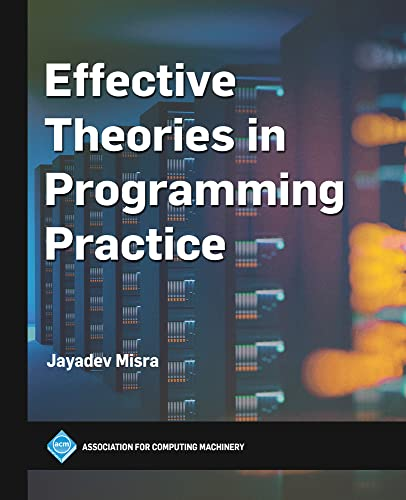# Effective Theories in Programming Practice

Description

Set theory, logic, discrete mathematics, and fundamental algorithms (along with their correctness and complexity analysis) will always remain useful for computing professionals and need to be understood by students who want to succeed. This textbook explains a number of those fundamental algorithms to programming students in a concise, yet precise, manner. The book includes the background material needed to understand the explanations and to develop such explanations for other algorithms. The author demonstrates that clarity and simplicity are achieved not by avoiding formalism, but by using it properly.

The book is self-contained, assuming only a background in high school mathematics and elementary program writing skills. It does not assume familiarity with any specific programming language. Starting with basic concepts of sets, functions, relations, logic, and proof techniques including induction, the necessary mathematical framework for reasoning about the correctness, termination and efficiency of programs is introduced with examples at each stage. The book contains the systematic development, from appropriate theories, of a variety of fundamental algorithms related to search, sorting, matching, graph-related problems, recursive programming methodology and dynamic programming techniques, culminating in parallel recursive structures.